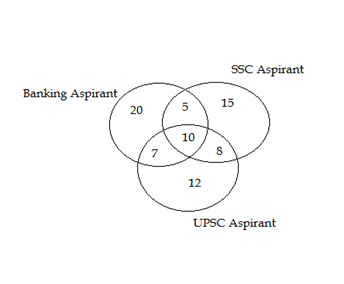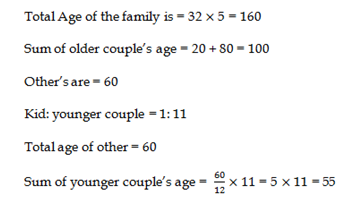# RRB ALP 2018 Practice Test Papers | Arithmetic Questions (Day-76)

Dear Aspirants, Here we have given the Important RRB ALP & Technicians Exam 2018 Practice Test Papers. Candidates those who are preparing for RRB ALP 2018 can practice these Arithmetic Questions to get more confidence to Crack RRB 2018 Examination.

[WpProQuiz 3065]

Click “Start Quiz” to attend these Questions and view Solutions

1) The ratio between Selling price and cost price of the article is 4: 3. Find the ratio of Profit and Cost price

a) 3: 1

b) 2: 3

c) 4: 5

d) 1: 3

2) In an exam consisting of 40 questionscarrying 2 marks each question, Shakshi Attempts 80%of questions correctly, how many wrong answers given by shakshi?

a) 4

b) 8

c) 12

d) 10

3) What will come in place of question mark (?) in the following question?

50% of 30 × 225 ÷27 =5?

a) 1

b) 2

c) 3

d) 4

4) 10 men can complete a work in y days. If 12 men can complete the ¾ of the work in 15 days, then y men can complete the work in how many days?

a) 14 days

b) 12 days

c) 16 days

d) 10 days

5) Average Ages of a family consists of 5 members are 32. There are 2 married couples and a kid in the family. Ratio of Kid and younger couple is 1 : 11. Sum of older couple’s age is 20 more than half of the family’s total age. Find the sum of younger couple’s age?

a) 60

b) 55

c) 50

d) 45

6) Find the mode of given numbers

2, 5, 6, 7, 2, 6, 9, 5, 8, 5, 3, 5, 3, 4, 6, 8, 9, 0, 3, 2, 4, 2, 2

a) 2

b) 8

c) 4

d) 9

7) A cyclist goes with a speed of 4kmph and return with the speed of 6 kmph. Find the average speed of the cyclist.

a) 3.6 Kmph

b) 4.2 kmph

c) 4.8 kmph

d) 3.2 kmph

Direction: (8 – 10) Read the instructions carefully and answer the question given below

In a competitive exams coaching institution, following diagram illustrates about student details8) How many aspirants are only preparing for banks exams?

a) 20

b) 5

c) 8

d) 12

9) What is the diffrence between number of  UPSC aspirant and SSC aspirant?

a) 0

b) 5

c) 4

d) 1

10) What is the total no. of aspirant  preparing for exams in the institution?

a) 33

b) 47

c) 65

d) 50

Selling price of the article = 4x

Cost price of the article = 3x

Profit = x

Ratio of Profit that of Cost price = 1:3

Total Question = 40

Total Marks = 80

Correctly attempted questions = 80*40/100 = 32

Wrong answers = 40 – 32 = 8

15×225 ÷ 27 = 15 × 225 × 1/27 = 125 = 53

12Men ———————- x Days                      1 Work
12Men ———————— 15 days             3/4 work

12x = 4×4×15 => x = 20

12  Men —————20 days

10 men ————— 24 days

Y=24

24 men—————-10 daysArrange the given data in ascending order

0, 2, 2, 2, 2, 2, 3, 3, 3, 4, 4, 5, 5, 5, 5, 6, 6, 6, 7, 8, 8, 9, 9

Here 2 occur more frequently in 5 times, so 2 is the mode of given data.

Average speed = 2(Speed 1 × Speed 2)/ sum of the speeds

= 2(4×6)/10 = 48/10 = 4.8 kmph

Only Banking aspirant = 20

No. of UPSC Aspirant = 7+8+10+12=37

No. of SSC Aspirant =  5+10+8+15=38

Difference = 38 – 37 = 1

7+8+10+12+5+10+8+15+20=95

RRB ALP 2018 – “All in One” Study Materials and Practice Sets